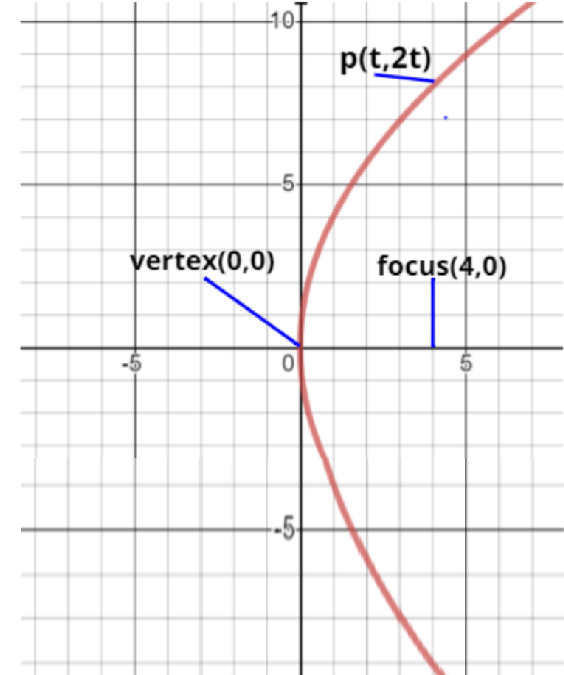QuestionAnswers

# The focal distance of a point on the parabola ${y^2} = 16x$ whose ordinate is twice as abscissa, a. 6b. 10c. 8d. 12Verified
163.2k+ views

Hint: Compare the given equation with the standard equation of parabola. Take a point P on parabola, and find out the coordinates of it. Now, by using distance formulas, find the focal length.

As, we know that the standard equation of parabola is $(y - {y_0})^2 = 4a(x - {x_0})$.

In which,

$\Rightarrow$ Vertex = $\left( {x{}_0,{y_0}} \right)$ and,

$\Rightarrow$ Focus = $\left( {{x_0} + a,{y_0}} \right)$

Given Equation of parabola is ${y^2} = 16x$

$\Rightarrow {y^2} = 16x$ - (Eq 1)

Comparing equation 1with standard equation of parabola we get,

$\Rightarrow$ ${x_0} = 0,{y_0} = 0$ and $a = 4$

So, focus of the equation 1 will be,

$\Rightarrow$ focus  = $\left( {4,0} \right)$

Let there be a point P on parabola, whose abscissa be t,

Then the ordinate of the point P will be 2t (According to question)

$\Rightarrow$ P = $\left( {{\text{t,2t}}} \right)$

According to the question point P lies on the parabola given (equation 1)

So, point P must satisfy equation 1

Putting value of P in equation 1 we get,

$\Rightarrow {(2t)^2} = 16(t)$

$\Rightarrow 4{t^2} - 16t = 0$

$\Rightarrow 4t(t - 4) = 0$

So, the value of t will be 4.

Hence point P = (4,8)

Now, as we know focal distance is the distance between focus and a point on a curve, focal distance will be the distance between focus and P.

Now, calculating distance between them as,

focal distance = $\sqrt {\left( {{{\left( {4 - 4} \right)}^2} + {{\left( {8 - 0} \right)}^2}} \right)} {\text{ }} = 8$

focal distance  = 8

Hence the correct option for the question will be (c)

NOTE: - Point on a conic always satisfies the equation of conic. Abscissa is the x - coordinate of a point and ordinate is the y - coordinate of a point.